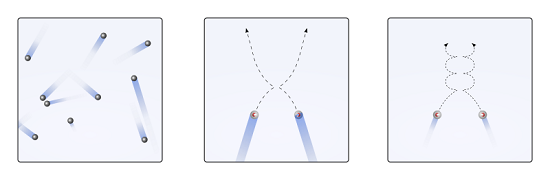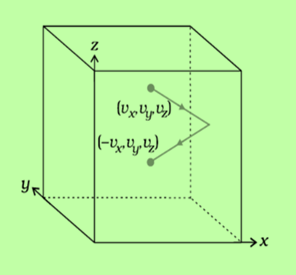# Kinetic Theory of Gases Assumptions

## Introduction

In our daily life, we commonly observe the motion of the air molecules when a light ray enters the dark through a small hole. the motion of air molecules is studied by the kinetic theory. With the help of the kinetic theory of various properties of the gas can be defined in terms of the pressure(P)and volume(V) as well as temperature(T) of the container where the gas is present. Tiny particles do not move in an upward or downward direction but they can move in all possible directions. We can’t see these particles as their size is very small but we see a movement of big particles.Olivier Cleynen and User:Sharayanan, Kinetic theory of gases (2), CC BY-SA 3.0

## What is the Kinetic Theory of Gases?

Gas contains a very large number of particles whose size is negligible known as molecules. These molecules are in a state of continuous or constant motion or in random motion. During this random motion, molecules continuously move with some velocity and the walls of the container or vessel where it is kept.

These collisions are perfectly elastic, almost instantaneous(time duration is small) and between the collision, molecules move in a straight line with acquiring uniform velocity.

## Assumption

• Particles are always continuously moving randomly in all directions but the path it covers is in a straight line.

• Particles are independent of any attractive or repulsive force.

• Gas is the collection of a large number of tiny particles having small mass like atoms or molecules

• The space between the particles is very large as the particles are far away from each other. so compared with the volume of the container, the volume of the particles is negligible.

• Particles are always in constant motion that's why they have kinetic energy which is directly proportional to temperature

• Due to collision between tiny particles or with containers, collision is perfectly elastic and does not change the momentum or energy of the particles. Pressure is also exerted on the container as particles collide with the container. This pressure depends on the number of particles colliding per unit area.

## Kinetic Energy and Pressure

As we know momentum is the multiplication of mass and velocity.Fig:2 motion of molecule in different components

Change in the momentum of the molecule

$\mathrm{\Delta P=P_2-P_1}$

$\mathrm{\Delta P=mv_x-(-mv_x)}$

$\mathrm{P=2mv_x}$

time for a consecutive strike $\mathrm{t=\frac{2A}{V_x}}$ So Force on the wall is given by

$\mathrm{F=\frac{\Delta P}{\Delta t}}$

$\mathrm{F=\frac{2mv_x}{2Av_x}}$

$\mathrm{F=m v_x^{2}}$

pressure in the x-direction, $\mathrm{P_x=\frac{F_x}{A}=\frac{m}{A^3}(v_x^{2})}$

Similarly for the y-direction $\mathrm{P_y=\frac{m}{A^3}(v_y^{2})}$

and for the z-direction, $\mathrm{P_z=\frac{m}{A^3}(v_z^{2})}$

According to pascal’s law,

$\mathrm{P_x=P_y=P_z=P}$

we can write, $\mathrm{P=\frac{P_x+P_y+P_z}{3}}$

Now putting all the direction of pressure,

$\mathrm{P=\frac{m}{3A^3}(v_x^{2})+\frac{m}{3A^3}(v_y^{2})+\frac{m}{3A^3}(v_x^{2})}$

$\mathrm{P=\frac{m}{3A^3}(v_x^{2}+v_y^{2}+{v_z}^2)}$

$\mathrm{P=\frac{m}{3A^3}(v_{RMS}^2)}$

also, we can write, $\mathrm{P=\frac{1}{3}\rho (v_{RMS}^2).......(1)}$

where $\mathrm{v_{RMS}}$ - Root mean square velocity

$\mathrm{\rho}$ - Density of the molecules

Kinetic Energy per unit volume,

$\mathrm{E=\frac{1}{2}\rho v^2...…(2)}$

Dividing eq (1) and (2)

$\mathrm{\frac{P}{E}=\frac{\frac{1}{3}\rho (v_{RMS}^2)}{\frac{1}{2} \rho v^2}=\frac{2}{3}}$

$\mathrm{P=\frac{1}{2}E}$

So pressure $\mathrm{=\frac{1}{2}}$ of Kinetic energy per unit volume

## Kinetic Energy and Temperature

According to kinetic theory

$\mathrm{P=\frac{1}{3}\rho (v_{RMS}^2)}$

$\mathrm{\rho=\frac{M}{v}}$

$\mathrm{P=\frac{1M}{3v}(v_{RMS}^2)}$

Multiply and divide by 2

$\mathrm{PV=\frac{2}{3}\frac{1}{2}(v_{RMS}^2)}$

$\mathrm{PV=\frac{2}{3}E}$

{As we know the ideal gas equation for 1-mole PV=RT}

$\mathrm{RT=\frac{2}{3}E}$

$\mathrm{E=\frac{3}{2}RT}$

Mean kinetic energy per molecules

$\mathrm{\overline{E}=\frac{E}{N}=\frac{3}{2}\frac{R}{N}T=\frac{3}{2}K_B T}$

## Relaxation Time

This is also known as mean free time. The motion of particles is completely random. During their motion, they collide and also change their direction. With each collision, they change their direction randomly.

So between two successive collisions, the average time or time interval between two particles is called the term relaxation time.

## Mean Free Path

Between two successive collisions, a molecule moves along a straight line path with uniform velocity. The average distance traveled or taken by the particle to meet the other particle. Not every path length or free path is the same. It may vary from different particles. It is represented as ‘λ’.

Mean free path,

$\mathrm{\lambda=\frac{1}{\sqrt{2}\pi d^2 \frac{N}{V}}(Where\:n=\frac{N}{V})}$

$\mathrm{\lambda=\frac{1}{\sqrt{2}\pi d^2 n}}$

Where n is the number of molecules per unit volume

d is the diameter of the molecule

## Brownian Motion

Images Coming soon

Fig:3 Brownian motion

If fine particles (atoms or molecules) are suspended(sprinkle) in any fluid either liquid or gas, they start doing random motion in all possible directions and collide with each other, and with the container, they keep and form a zig-zag path. This motion is called Brownian motion.

Robert Brown, the botanist described this motion first in 1827 and thus for honor, this motion is named Brownian motion while looking at pollen grains that are immersed in liquid through a microscope.

Later in 1905, Einstein came up with his theory of Brownian motion. Pollen grains move randomly due to collision or bombardment of the particles. This is what einstein showed in his paper. Jean Perrin confirmed the explanation and got the Nobel Prize in 1926 for it. Some behavior can be observed in the sunlight coming into a dusty room. We observed dust particles moving randomly.

## Conclusion

The kinetic theory of gas is very helpful in understanding the macroscopic properties or microscopic properties of the particles. By knowing temperature we can easily find average kinetic energy as kinetic energy is directly proportional to temperature. All these can be studied in atomic theory. The kinetic theory of gas concludes that the particles are always in motion.

## FAQs

Q1. What are the quantities the root mean square molecular speed depends on?

Ans. As we know, $\mathrm{v_{RMS}=\frac{3RT}{M}}$

The velocity of the gas is

• Directly proportional to absolute temperature

• Inversely it proportional to the molar mass of the gas

also, $\mathrm{Molar\:mass=\frac{Volume}{mole}}$

$\mathrm{Molar\:mass=\frac{Volume}{\frac{mass}{M}}}$

Therefore molar volume is directly proportional to the root mean square velocity of the gas.

Q2. A truck with goods moving with uniform velocity. What will be the temperature of the gas molecules inside the cooking gas when kept in the truck?

Ans. The molecules attain relative velocities and the root means square velocities are different from lateral translation.

Q3. Which factors on which the average kinetic energy of gas molecules depends?

Ans. The average kinetic energy of a gas atom or molecule is dependent on temperature as kinetic energy is directly proportional to absolute temperature.

$\mathrm{K=\frac{3}{2}\frac{R}{N_A}T}$

Q4. If the volume of hydrogen and oxygen samples are the same then state that which has the larger number of molecules?

Ans. If the volume of the two samples is the same, the temperature and pressure of gases are the same. As a result, the molecules of both the samples are the same.

Q5. What will be the temperature, root mean square velocity of the hydrogen(H) molecule comes equal to the oxygen(O) molecule at 79°.

Ans. As we know,

$\mathrm{v_{RMS}=\sqrt{\frac{RT}{M}}}$

The root means square velocity of an oxygen molecule is equal to the mean square velocity of hydrogen.

$\mathrm{\sqrt{\frac{273+79}{32}}=\sqrt{\frac{T}{2}}}$

$\mathrm{\frac{352}{32}=\frac{T}{2}}$

$\mathrm{11\:\times\:2=T}$

$\mathrm{T=22^{\circ}C}$

Therefore 22°C temperature is required.